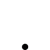Voltage

• Topic Is Sticky
• 998 Views
• Last Post 4 weeks ago
Chris posted this 08 March 2020

My Friends,

Be it known, I am still working in this area, for some this might be easy, for me, this is not so easy.

I have deliberately left this topic last, for a very good reason! Voltage, what is Voltage and how is it "Generated"?

Bucking Coils Voltage Requirement!

From the early days, we have had problems with Voltage! At one point I asked the question in one of my Mr Preva Experiments, why such a large Voltage Drop?

In a Transformer the Voltage is given by:

VRMS = 4.44 N f ΦPeak A 10-8

Floyd Sweet told us, straight up:Note: My research led me to believe Floyd Sweet was fond of Peter Bruce, and I believe if anyone has the full, true story on the VTA, it will be Peter Bruce, but I have never been able to get hold of him.

Which is a rearrangement of the above Transformer Equation, to get Turns instead of Volts rms.

Floyd Sweet Defined Voltage like so:

Voltage: The energy transfer capability of flow of electric charge is determined by the potential difference or voltage through which the charge moves. A charge of 1 coulomb receives or delivers an energy of one joule or watt-second in moving through a voltage of 1 volt or V = dw / da.

For my poor old brain, some studding to understand that! The Charge Potential Difference between two Terminals, Coulombs of Potential.

Even in The Mr Preva Experiment, we have all had trouble with getting the Voltage up, it sounds like a simple task, but it is not as easy as one would thing!

We know, Floyd Sweet especially, worked hard on increasing the Cross Sectional Area of his Coils so he could have less turns of a thicker wire gauge, we have Visual evidence and also Lab Note Evidence for this.

Now, our Partnered Output Coils:I pointed out the Turns relationship on the VTA Thread between the Coils:

On the Topic and Point of Transformers and Floyd Sweet being a Transformer Expert, What are the turns Ratio we see: 1 : 5 = 48 Turns to 240 turns! So 240 / 48 = 5 right?

Ok, another point no one has ever bought up: 120 Volts / 240 Turns = ‭0.5‬ Volts per turn. 48 * 0.5 =  24 Volts, what did we use to start the VTA? 2 - 15 Volts right. So whats the chances the Turns Ratio are so close?

A Transformer? Yes of course the VTA is a Transformer variant!

We will always have those with their own opinion, however, an Educated Opinion has worth, an uneducated opinion is worthless! Beware those Un-Educated opinions! They will steer you down all sorts of Rabbit Holes!

The VTA was, plain and simple, a Generator and a Transformer all in one!

If the Gold Coil on the Left, POCOne, has a Sinusoidal Current of 1.0 Amperes at 50 Hz, to "Generate" a Voltage in the Right Gold Coil, POCTwo, of 20 Volts, then what else do we need to know? Length = 0.03M, 9 Turns, Permeability = 1000, now this gives us, B = 3769.9111843 Gauss or 0.3769911 Tesla.

So, if we plug this into our Faraday's Law Calculator:

• Area: 0.121922069293166 Meters (m) Squared or 12.1922069293166 cm squared.
• Theta: 90 Degrees
• B Initial: 0.0001 Tesla
• B Final: 0.37699111843 Tesla
• Delta B: 0.413562105514813
• Delta T: 0.02 Seconds
• Voltage: 20.6781052757406 Volts
• Amperes: 6.89270175858021 Amperes

Of course, this does not work out in the way we have tried to work out, if we try this, build it, configure the right Feed Forward and Feed Back Currents, we don't get this as we would expect. Why? Whats stopping us? Why cant we make this what should be a simple calculation?

The Aboveunity.com Member Calculator can be used to calculate the B Field, and the following class can be used to calculate Electromagnetic induction:

///////////////////////////////////////////////////////////////////////////////////////////////////////////////////////////
///   ****************************************************************************************************************   //
///   Written by Chris Sykes                                                                                             //
///   Copyrght 2016                                                                                                      //
///   --> Application Name Goes Here <--                                                                                 //
///                                                                                                                      //
///////////////////////////////////////////////////////////////////////////////////////////////////////////////////////////
namespace EScience
{

#region Using Statements:

using System;

#endregion

{

#region References:

// See: http://farside.ph.utexas.edu/teaching/302l/lectures/node95.html

/*

* A plane circular loop of conducting wire of radius $r=10$cm which possesses $N=15$ turns is placed in a uniform magnetic field.
* The direction of the magnetic field makes an angle of $30^\circ$ with respect to the normal direction to the loop.
* The magnetic field-strength $B$ is increased at a constant rate from $B_1=1$T to $B_2=5$T in a time interval of  ${\mit\Delta}t=10$s.

* What is the emf generated around the loop?
* If the electrical resistance of the loop is $R=15\,\Omega$, what current flows around the loop as the magnetic field is increased?
*
*

* The area of the loop is  -  \begin{displaymath} A = \pi\,r^2 = \pi\,(0.1)^2 = 0.0314\,{\rm m}^2. \end{displaymath}

* The component of the magnetic field perpendicular to the loop is - \begin{displaymath} B_\perp = B\,\cos\theta = B\,\cos 30^\circ = 0.8660\,B, \end{displaymath}

* Where $B$ is the magnetic field-strength.

* Thus, the initial magnetic flux linking the loop is - \begin{displaymath} {\mit\Phi}_{B\,1} = N\,A\,B_1\,\cos\theta = (15)\,(0.0314)\,(1)\,(0.8660) = 0.408\,{\rm Wb}. \end{displaymath}

* Likewise, the final flux linking the loop is - \begin{displaymath} {\mit\Phi}_{B\,2} = N\,A\,B_2\,\cos\theta = (15)\,(0.0314)\,(5)\,(0.8660) = 2.039\,{\rm Wb}. \end{displaymath}

* The time rate of change of the flux is - \begin{displaymath} \frac{d{\mit\Phi}_B}{dt} = \frac{{\mit\Phi}_{B\,2}- {\mit\P... ...Delta}t} = \frac{(2.039-0.408)}{(10)}=0.163\,{\rm Wb\,s}^{-1}. \end{displaymath}

* Thus, the emf generated around the loop is - \begin{displaymath} {\cal E} = \frac{d{\mit\Phi}_B}{dt} = 0.163\,{\rm V}. \end{displaymath}

* Note, incidentally, that one weber per second is equivalent to one volt.

* According to Ohm's law, the current which flows around the loop in response to the emf is - \begin{displaymath} I = \frac{{\cal E}}{R} = \frac{(0.163)}{(15)} = 0.011\,{\rm A}. \end{displaymath}

*/

#endregion

#region Fields:

// Magnetic Field B specified in the Constructor:
private double B;

#endregion

#region Properties:

/// <summary>
/// Cross Sectional Area in Meters Squared.
/// </summary>
public double A
{
get;
set;
}

/// <summary>
/// Ohms Law can calculate the Amperes.
/// Calculated as: I = V / R
/// </summary>
public double Amperes
{
get;
set;
}

/// <summary>
/// Initial B
/// Calculated as: B = μNI/L
/// </summary>
public double BInitial
{
get;
set;
}

/// <summary>
/// Final B
/// Calculated as: B = μNI/L
/// </summary>
public double BFinal
{
get;
set;
}

/// <summary>
/// Total Change in Magnetic Field B, through Area A.
/// Calculated as: BFinal - BInitial = DeltaB
/// </summary>
public double DeltaB
{
get;
set;
}

/// <summary>
/// The Time Rate of Change of the Magnetic Flux in Seconds.
/// </summary>
public double DeltaT
{
get;
set;
}

/// <summary>
/// The Initial Magnetic Flux Linking the Loop.
/// Calculated as: N A BInitial Cos(Theta)
/// This Value is in Webbers.
/// </summary>
public double PhiB1
{
get;
set;
}

/// <summary>
/// The Final Magnetic Flux Linking the Loop.
/// Calculated as: N A BFinal Cos(Theta)
/// This Value is in Webbers.
/// </summary>
public double PhiB2
{
get;
set;
}

/// <summary>
/// The Resistance (R) of the loop of wire.
/// </summary>
public double R
{
get;
set;
}

/// <summary>
/// Theta (&theta the Angle of the Magnetic Flux.
/// This is the Angle away from Perpendicular (90 Degrees) from the Plane of the Conductor.
/// </summary>
public double Theta
{
get;
set;
}

/// <summary>
/// The Turns of the Conductor.
/// </summary>
public double Turns
{
get;
set;
}

/// <summary>
/// Time Rate of Change of the Flux in Webbers per second.
/// One Webber per second is equivilent to One Volt.
/// </summary>
public double Wbs
{
get;
set;
}

/// <summary>
/// The Total E.M.F (Coulombs of Charge) measured in Volts.
/// One Webber per second is equivilent to One Volt.
/// 1 C is equal to approximately 6.24 x 10^18, or 6.24 quintillion
/// </summary>
public double Volts
{
get;
set;
}

#endregion

/// <summary>
/// Initialises a new instance of Faraday's Law of Electromagnetic Induction.
/// </summary>
/// <param name="NumberOfTurns">The number of Turns of the Conductor</param>
/// <param name="Theta">The Angle, Perpendicular to the Plane of the Conductor in Degrees. E.G: If the Flux is at 90 Degrees, Theta will be: 0</param>
/// <param name="BInitial">The Initial Flux Linking the Loop, often this will be Zero in Units of Tesla</param>
/// <param name="BFinal">The Maximum Magnetic Field in Units of Tesla</param>
/// <param name="DeltaT">The Time Rate of Change in Seconds - Frequency or Cycles per Second</param>
/// <param name="Resistance">The Resistance of the Conductor</param>
public FaradaysLaw(double Radius, double NumberOfTurns, double Theta, double BInitial, double BFinal, double DeltaT, double Resistance)
{

this.Turns = NumberOfTurns;
this.BInitial = (BInitial == 0 ? 1 : BInitial);
this.BFinal = BFinal;
this.DeltaT = DeltaT;
this.B = this.BInitial;
this.R = Resistance;

CalculatePhiB1();

CalculatePhiB2();

CalculateDeltaB();

CalculateWebbersAndVolts();

CalculateAmperes();
}

/// <summary>
/// Initialises a new instance of Faraday's Law of Electromagnetic Induction.
/// </summary>
/// <param name="Length">Length of the Conductor in Centimeters</param>
/// <param name="Width">Width of the Conductor in Centimeters</param>
/// <param name="NumberOfTurns">The number of Turns in the Conductor</param>
/// <param name="Theta">The Angle, Perpendicular to the Plane of the Conductor in Degrees. E.G: If the Flux is at 90 Degrees, Theta will be: 0</param>
/// <param name="BInitial">The Initial Flux Linking the Loop, often this will be Zero in Units of Tesla</param>
/// <param name="BFinal">The Maximum Magnetic Field in Units of Tesla</param>
/// <param name="DeltaT">The Time Rate of Change in Seconds - Frequency or Cycles per Second</param>
/// <param name="Resistance">The Resistance of the Conductor</param>
public FaradaysLaw(double Length, double Width, double NumberOfTurns, double Theta, double BInitial, double BFinal, double DeltaT, double Resistance)
{

CalculateArea(Length, Width);

this.Turns = NumberOfTurns;
this.BInitial = (BInitial == 0 ? 1 : BInitial);
this.BFinal = BFinal;
this.DeltaT = DeltaT;
this.B = this.BInitial;
this.R = Resistance;

CalculatePhiB1();

CalculatePhiB2();

CalculateDeltaB();

CalculateWebbersAndVolts();

CalculateAmperes();
}

/// <summary>
/// Calculate the Area of a Circle:
/// </summary>
{

// Circumference is:
// C = 2 * Math.PI * Radius

// Area of a Circle is:
this.A = (Math.PI * Math.Pow(Radius, 2)) / 100;
}

/// <summary>
/// Calculate the Area of a Rectangle, or a Square from L x W.
/// </summary>
/// <param name="Length">Length of the Rectangle or Square</param>
/// <param name="Width">Width of the Rectangle or Square</param>
private void CalculateArea(double Length, double Width)
{
// Length x Width is:
this.A = (Length * Width) / 100;
}

/// <summary>
/// Calculate PhiB1. This Value is in Webbers.
/// </summary>
private void CalculatePhiB1()
{
this.PhiB1 = this.Turns * this.A * this.BInitial * Math.Cos(this.Theta);
}

/// <summary>
/// Calculate PhiB2. This Value is in Webbers.
/// </summary>
private void CalculatePhiB2()
{
this.PhiB2 = this.Turns * this.A * this.BFinal * Math.Cos(this.Theta);
}

/// <summary>
/// Calculate DeltaB from the Constructor Input.
/// </summary>
private void CalculateDeltaB()
{

this.DeltaB = this.PhiB2 - this.PhiB1;
}

/// <summary>
/// Calculate the Webbers and Volts all at once, they are the same.
/// </summary>
private void CalculateWebbersAndVolts()
{

this.Wbs = this.DeltaB / this.DeltaT;

this.Volts = this.Wbs;
}

/// <summary>
/// Calculate the Amperes that the Conductor can Supply.
/// </summary>
private void CalculateAmperes()
{
this.Amperes = this.Volts / this.R;
}

} // END of Class...

} // END of Namespace...

I mean, this basic idea works in a Generator, or a Transformer, why is this not working for us? The configuration is exactly the same is it not? Whats different? What are we missing?

The answer to these questions, the solution to this Voltage issue as you all know is not plain and simple, I hope our excellent team can work on this together!

Chris

Forelle posted this 08 March 2020

Hi everyone,

in regard to Chris's question

"I mean, this basic idea works in a Generator, or a Transformer, why is this not working for us? The configuration is exactly the same is it not? Whats different? What are we missing?"

I am asking this myself all the time, i think i should start at the simplest configuration in terms of parts and this is L1 L2 L3 with air core like a Don Smith device.I "only" want to get the waveforms and phasing right.It should also work with lower voltage.

Have a good day.

Ourbobby posted this 19 March 2020

Hi Chris,
This voltage topic thread could become a philosophical topic? The problem for me has also been expressd in another thread, which elludes me at the moment, regarding calculations to determine a potential. Ohms law is a legacy calculation based upon DC calculations, and power calculations. I2xR, V/R V/I etc. Ohms law appears to work with AC. But, only at low frequencies, so therefore it is marginally an AC calculation. As soon as we get into higher frequencies, Ohms law collapses. This is because the current is unable to travel without interference to suppose the Ohms Law condition. Thus Voltage is seen to be independent to current at higher frequencies. At low frequency, there appears to be a rigid association. But what if, there is a time differential between the accociation of current and voltage that is imperceptible at the fundamental low frequency?

Voltage is then either the precursor to current or the precursive of current. This is an interesting conundrum and one which most likely is not new. As it is seen that current has velocity and spin, then is voltage a manifestation of the spin phenomenon first? Or is is that the static environment we live in creates the situation for the electron spin? If so, then this is that which we seek. Too much for me at the moment. But, nevertheless, a useful pursuit!

Regards

ourbobby

Chris posted this 19 March 2020

Hi Ourbobby,

Your'e right, this topic could become a philosophical topic. We must force ourselves to stick to facts, use logic and reason with what we have. Lets use Floyd Sweet as an example, the Generation One VTA used Slab Magnets of 100mm x 150mm x 25mm: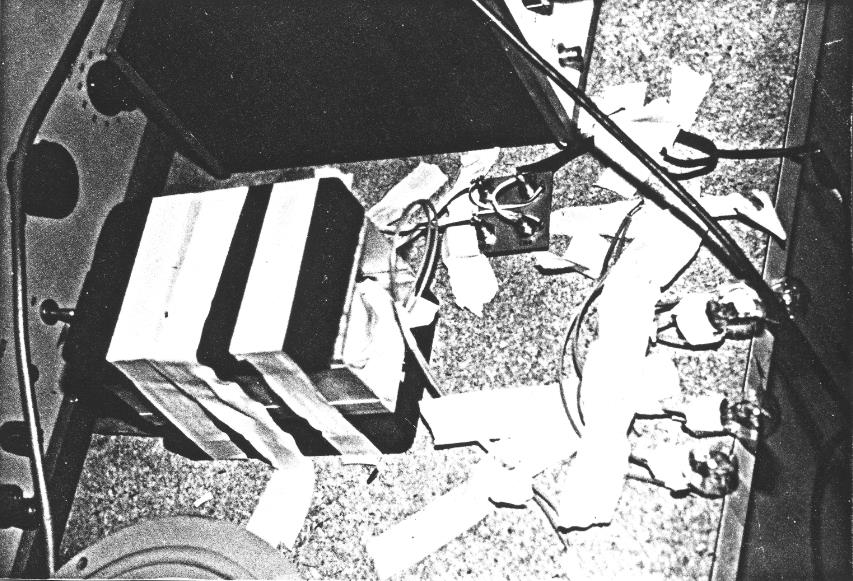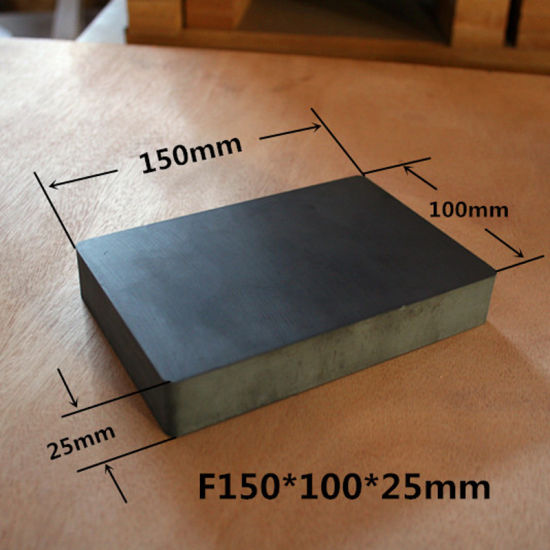However it was Generation Three before Floyd Sweet got a solid 120 Volts AC at 60 Hz that we can verify:The Magnet size was the same, but the depth was 12.5mm instead of 25mm. The Magnet is the same size: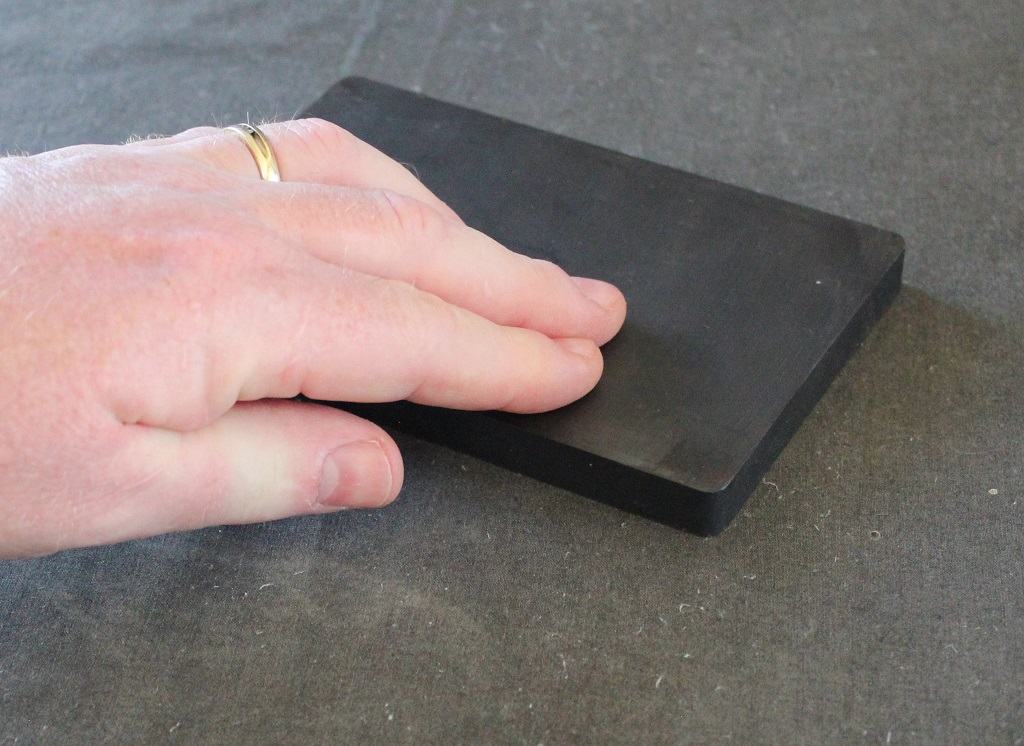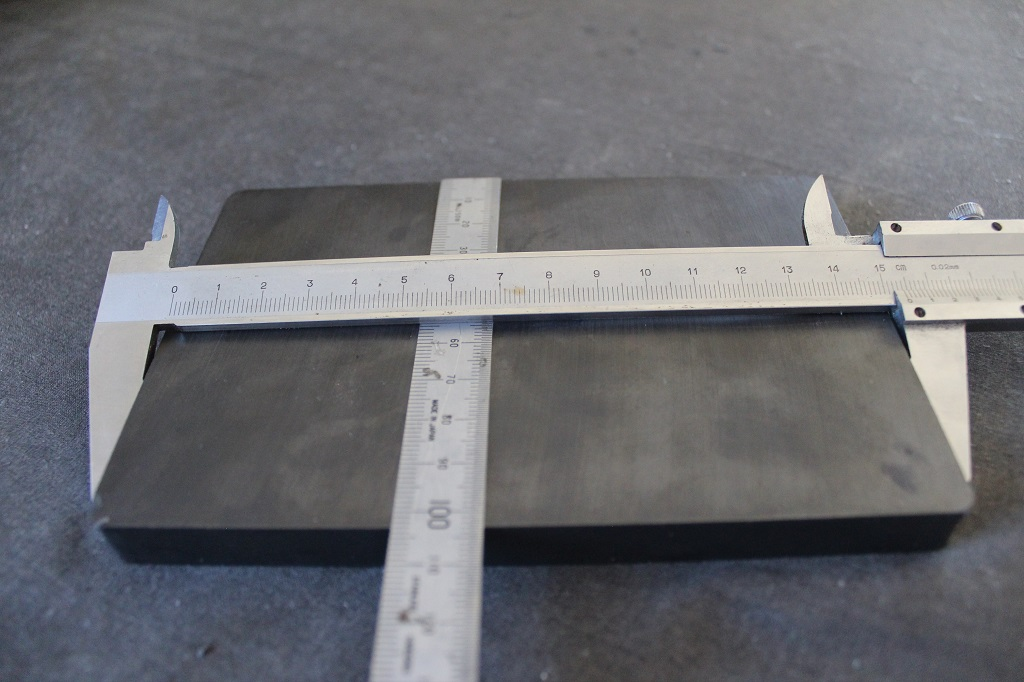The Magnets I have measured are mostly around the 700 to 800 Gauss, uniform across the face of the Magnet.

It is important to use facts, in Generation Five we know for sure, not sure on Generation Three, that Floyd Sweet used two sets of Power Coils, for example, the Schematic matched the geometry: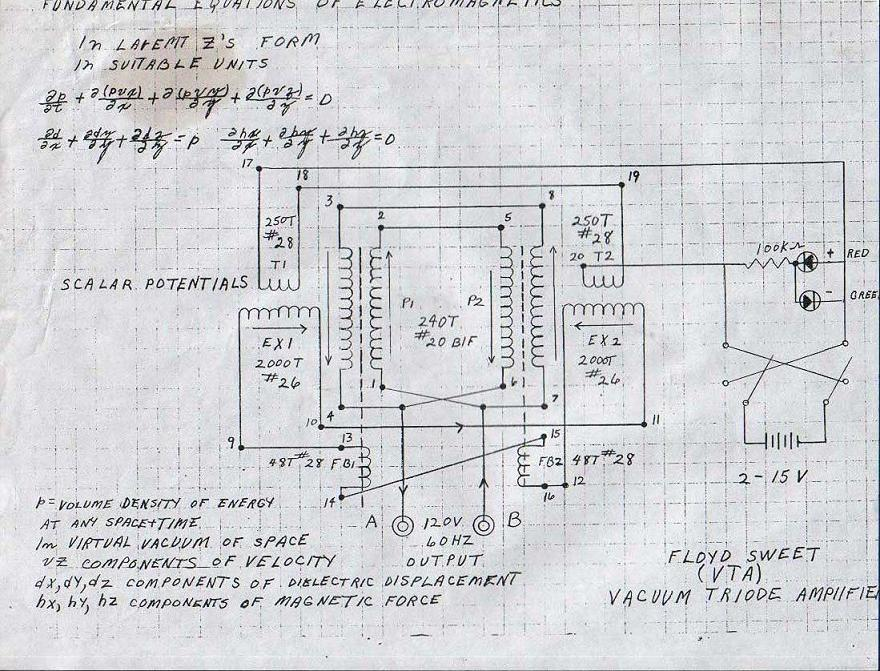This evidence gives us a fact we can verify, 120 Volts RMS at 60Hz, so how much Area, vs Flux Movement vs Turns do we need?

It turns out we have an almost perfect fit, using the Aboveunity Member Calculator: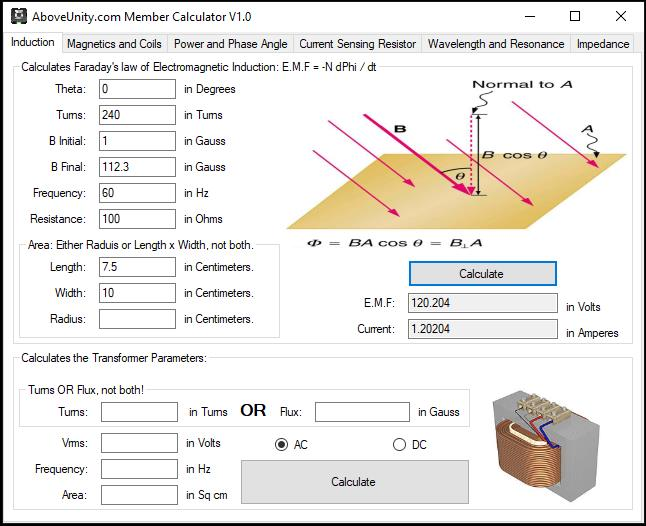Where:

• Theta = 0.
• Turns = 240.
• B-Initial = 1.
• B-Final = 112.3.
• Frequency = 60 Hz.
• Resistance = 100 Ohms.

Note: I used exactly half the Face of the Magnet for the Area, 7.5 x 10 = ‭75‬ Square Centimeters Each Coil, which the Coil will be less, but I wanted to show you close as possible to the above geometry as possible, gives us 120 Volts RMS with only 112.3 Gauss movement! This is a super achievable task, pleanty of room for margin here!

So, Facts are here to help us!

Floyd Sweet must have found a way to "Generate" sufficient Voltage, and thus then Pump Current. It is worth noting at this stage, the Sine wave Floyd Sweet showed us is odd:Ref: @ 26 : 04 - YT watch?v=zRd6lnKDLbE

The top of the Sine Wave is round as it should be, the the sides are Linear, not how a true sine wave should be!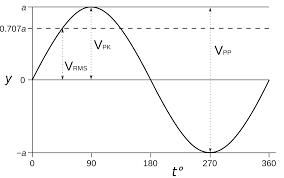The point is, Voltage is a base requirement! The Output, Real Power requires Voltage and therefore Current, in phase, together, is Real Power.

So Floyd Sweet found a way, to make Bucking Coils "Generate" enough Voltage in his VTA or SQM if you like the original term, Space Quanta Modulator. Voltage is a very important operator in our equations! We must spend more time here on this aspect!

Best wishes,

Chris

Chris posted this 20 March 2020

My Friends,

Voltage is a quantity of Charge:

Voltage, electric potential difference, electric pressure or electric tension is the difference in electric potential between two points. The difference in electric potential between two points in a static electric field is defined as the work needed per unit of charge to move a test charge between the two points. Wikipedia

Common symbols: V, ∆V, U, ∆U

Derivations from other quantities: Voltage = Energy / charge

Dimension: M L2 T−3 I−1

Formula, Ohm's law: V = IR

V = Voltage
I = Current
R = Resistance

Many website have definitions:

One volt will drive one coulomb ( 6.24 x 1018 ) charge carriers, such as electrons, through a resistance of one ohm in one second.

So why Voltage x Current? Well as pointed out, Current is 6.24 x 1018 Coulombs of Charge, past point p in one second. It may be obvious at this point, the Coulomb of Charge has a static value, and we can have many Coulombs of Charge. The Volt is a regulator allowing for the regulation of Coulombs of Charge flowing in One Second.

To increase the Coulombs of Charge flowing, we must increase the Voltage, the Potential Difference of the Charge. Voltage is the opening or closing of the Tap. Remember our threads have touched on the Volumetric Flow Rate, well, the same basic ideas apply. Volumetric flow rate can be defined by:

Q = v ⋅ A

Where:

• v = Flow Velocity
• A = Cross-Sectional Vector Area / Surface

We could use the analogy where, Voltage is the same as A, the Cross-Sectional Vector Area / Surface, and Current already has Flow Velocity, v. This is 6.24 x 1018 Coulombs of Charge per second.

Magnetic Flux and Voltage have a very close relationship!

The Weber, a unit of magnetic flux in the International System of Units ( SI ), defined as the amount of flux that, linking an electrical circuit of one turn ( one loop of wire ), produces in it an electromotive force of one volt as the flux is reduced to zero at a uniform rate in one second.

NOTE: Consider a single sinusoidal magnetic flux wave wave of peak amplitude Φ and frequency f. The flux will change from to in ½ f seconds. Average rate of change of flux = 2Φ ÷ 1/2f = 4fΦ Webers per second. 1 Weber per second = 1 Volt and noting that 1Wb = 108 lines of Flux. Average E.M.F. induced per turn = 4fΦ volts and the R.M.S. value is 1.11 times the average value.

In a Transformer, when dealing with the equations, we see the value of 1 Weber used all the time:

VRMS = 4.44 N f Φ A 10-8

or to calculate the turns:

N = VRMS 108 / 4.44 f Φ A

or to calculate the Flux:

Φ = VRMS 108 / 4.44 N f A

Where:

• 108 lines of Flux = 1 Weber per second.
• Φ = Flux.
• VRMS = Voltage RMS.
• 4.44 = Form Factor for AC.
• f = Frequency.
• A = Cross-Sectional Area.
• N = Turns.

I would like to stress, 108 lines of Flux changing in 1 Second Induces 1 Volt. So 1 Weber per second Induces 1 Volt in the corresponding Coil. How do we step forward with this logic?

Best wishes,

Chris

Jagau posted this 20 March 2020

Hi Chris

The formula I have in my book to find the rms voltage of a transformer is: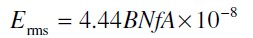B, flux density is in gauss and A is the area in squared centimiter that why we have at the end 10-8

if we were in weber by squared meter no need of 10-8 at the end.

It's like you said the 4 quadrant of a single sine wave multiply by the form factor 1.11 gives us 4.44

but  If we want only have average voltage we mulitply his entire formula by 1.11 as FLOYD sweet did in his formula and we come back to average voltage.

Floyd Sweet often wanted to shuffle the cards maybe that is what he meant when talking about average voltage ??

it's just my point of view.

Jagau

Chris posted this 20 March 2020

Hey Jagau,

Thank You for picking up and pointing our my error! Looking around, there are a few equations giving the Voltage RMS on the Coil. Not many explain why the Area and the 10-8 is used as you have! Thank You!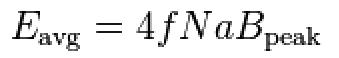Thank You for pointing out my error!

Yes, your'e right, Floyd Sweet was a little light on solid Facts! Its a shame! However, even though he did not like to provide Facts, we still can gain a lot of facts just by observation and common sense, as you point out. We know Voltage is a requirement on the Output, we know this to be factual. We know a Voltage is required for a Current to Flow, Ohms Law tells us this: V = IR.

So, even if Floyd Sweet did not place many facts into the public domain, we can still use what we know to be true and factual to give us other facts, for example, the VTA must have "Generated" a sinusoidal 120 Volts rms, we saw it on the scope.

Best wishes,

Chris

Ourbobby posted this 20 March 2020

Hello,

If you please be a little patient with me. Voltage must be a constant, and a variable constant when required. I have see a few comments explaining voltage through the use of associated formulae. Where, the associated formulae contains other contributors! I also sometimes get the impression I am looking at the chicken and egg conundrum: which was once explained to me, by a theoretical physicist as a ludicrous argument. I suppose, understanding where the source emanates, would give a clearer picture to useful knowledge. I understand that this thread is not really about determining the origin of voltage, but, its usefulness with the topics and experiments to hand.  I can get sidetracked trying to get to the fundamental proposition of exercises that I get involved with! So, at the moment, for me voltage is a pressure that affects or manipulates other conditions.

regards

ourbobby

Chris posted this 20 March 2020

Hi Ourbobby,

No, Voltage is not Constant, Current is not Constant either!

Perhaps you mean something else? The term Constant means: not changing, for eternity the same value. I can only guess you mean something else?

Of course, these machines require Change, implying the exact opposite of Constant!

Ohms Law is your friend! If there is no Voltage, then no Current can flow. I = V / R, V = IR, if Voltage = 0, then Current is also 0.

We truly rely on a Dynamic Machine, the change in Time, of Flux, Voltage and there fore Current. Nothing here is Constant.

Yes, when you say: "for me voltage is a pressure that affects or manipulates other conditions" this is one way to think of it.

Best wishes,

Chris

dale morgan posted this 20 March 2020

Forgot.… The only thing in the universe that is constant is Change.

Chris posted this 21 March 2020

My Friends,

We know for a fact Voltage on Bucking Coils is important!

I posted here:

I urge all here, study this video:

What does Graham show at minute:  4 : 54 on.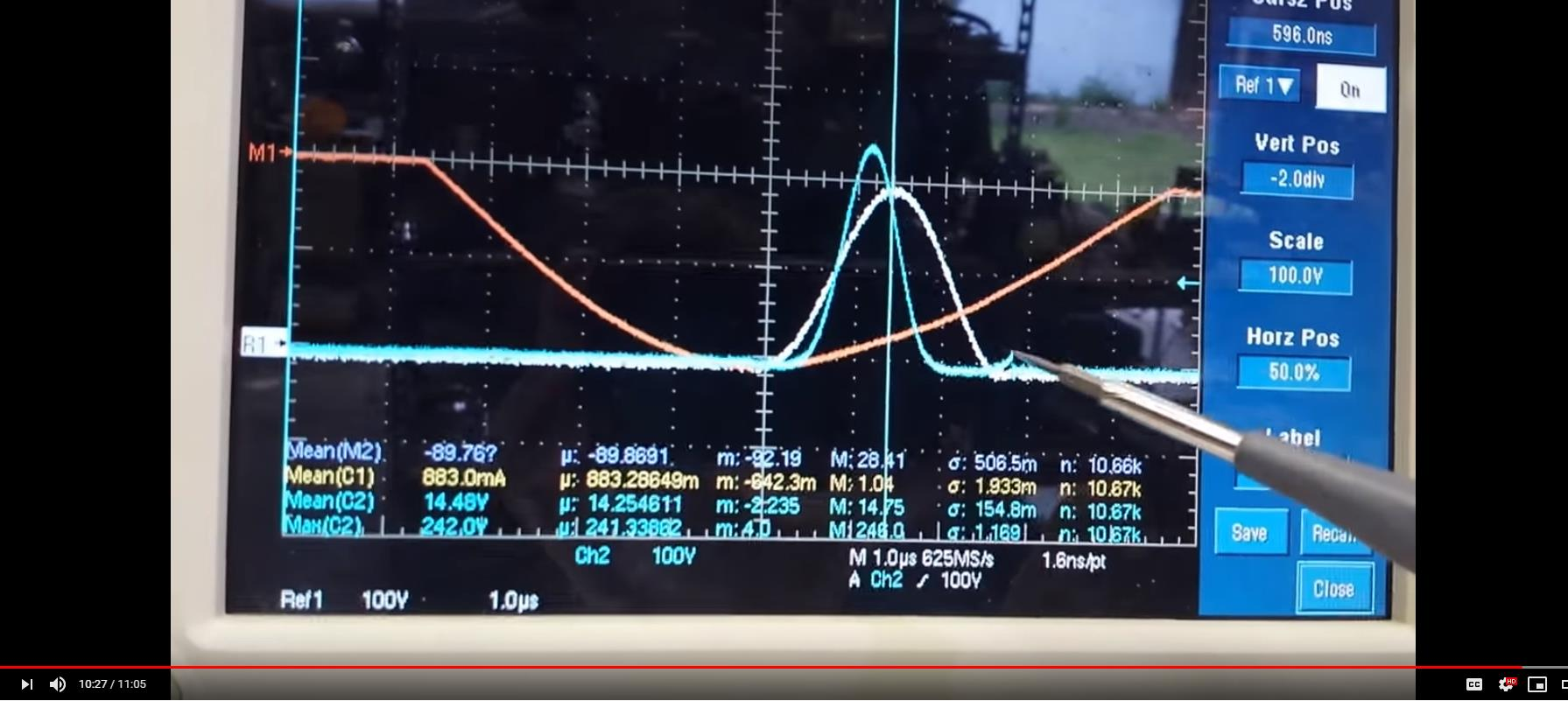Ref: @ 10 : 27

NOTICE: The Scope is 100 Volts per division.When Graham removed the Capacitors, the pulse was around: 200 Volts, when the Capacitors were removed, the Voltage was around 240 Volts. The sharper the Spike, the more E.M.F was Generated, thus the machine went Above Unity when more E.M.F was generated.

For this experiment, I added, a capacitor on each one of the Output Coils.

...

instead of a pulse width of about a micro second we have about 2 and a half microseconds.

Remember the difference: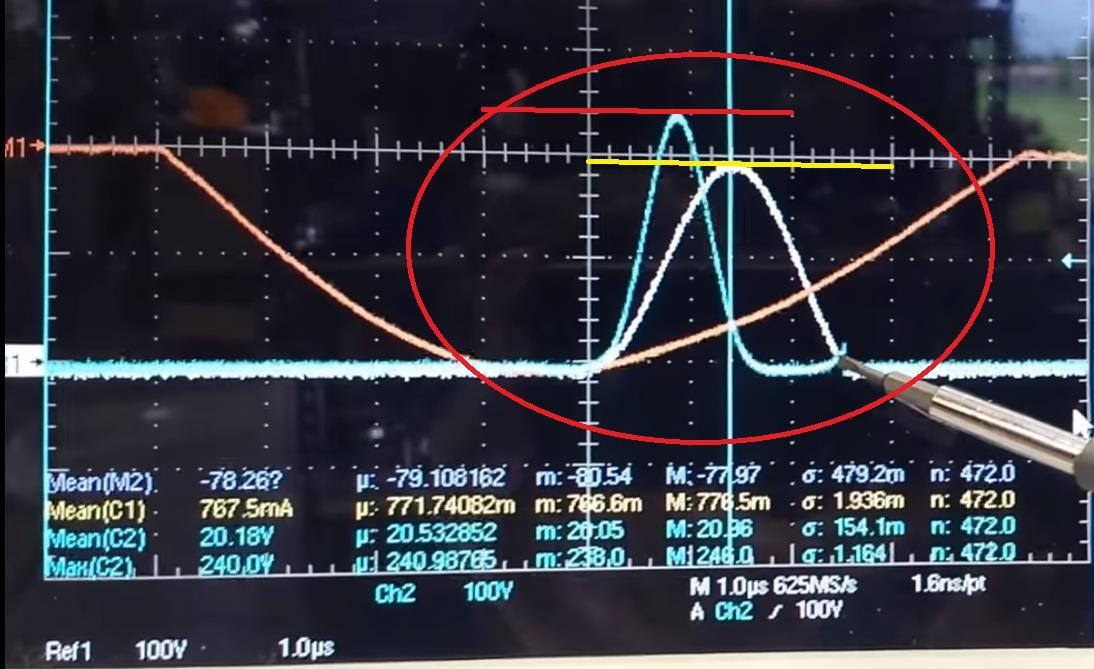The difference: 0.5 Watts Input 9.5 Watts Out vs 8.0 Watts Input 10.8 Watts Out

Now, remember, Ohms Law: I = V / R, Increase the Voltage, the Current is also increased for the same Resistance. The Potential Problem also pointed out by Tom Bearden:

White Pulse

@100 Volts per division, we have an EMF of 190 Volts approximately. At 190 Volts EMF, this gives us approximately 8.0 Watts Input 10.8 Watts Output. COP = 1.35‬.

We have a COP to EMF ratio of: ‭1.35‬ / 190 = ‭0.0071.

We have a EMF to Input Ratio of: 8.0 / 190 = ‭0.0421‬.

We have a EMF to Output Ratio of: 10.8 / 190 = 0.0568‬.

Blue Pulse

@100 Volts per division, we have an EMF of 240 Volts approximately. At 240 Volts EMF, this gives us approximately 0.5 Watts Input 9.5 Watts Output. COP = 19.

We have a COP to EMF ratio of: ‭19 / 240 = ‭‭0.0792.

We have a EMF to Input Ratio of: 8.0 / 240 = ‭‭0.0333‬‬.

We have a EMF to Output Ratio of: 9.5 / 240 = ‭0.0396‬.

All interesting numbers! We are seeing something that many would not think about! The COP to EMF Ratio is perhaps the most interesting! We can see a clear difference! As COP increases with the EMF we see a clear overall increase.

Best wishes,

Chris

Chris posted this 05 October 2020

My Friends,

We have seen, the Change in the Magnetic Field B, regulates the Voltage V.

We have seen, this is how a Common Made Choke works.

I posted here:

My Friends,

In Floyd Sweets VTA, I am fairly confident he used a simple Faraday's Law Equation to get his Voltage up, the task we have a bit of trouble with, but know of a few ways.

Remember what Walt Rosenthal told us:

The VTA "likes" to always see a minimum load of 25 watts.

Well, each Coil has a given Current, at 25 watts, and 120 Volts, the current is: 0.20833 Amperes.

Of course, knowing the Coil Dimensions, one could calculate the Magnetic Field B: 62.8308478 Gauss.

Note: Again I am speculating some what, I am not intending to mislead anyone but want to share some basics: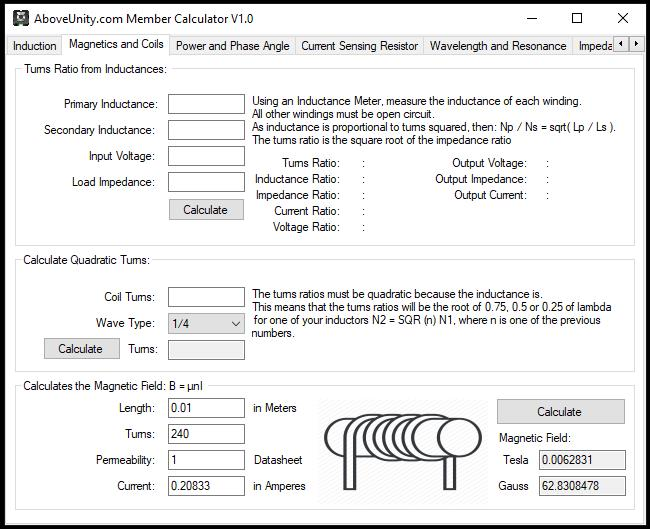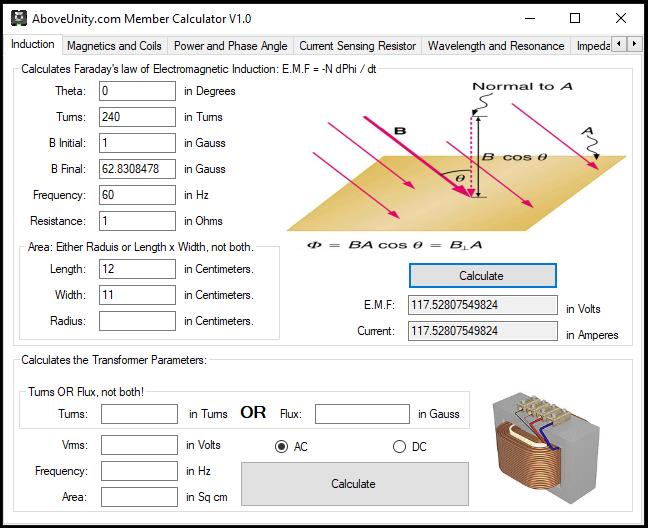Consider for a moment the construction of the triode which includes the bifilar coils located within the fields of the two conditioned magnets.

When the current in one half of the conductors in the coils (i.e., one of the bifilar elements in each coil) of the device is moving up, both the current and the magnetic field follow the right-hand rule.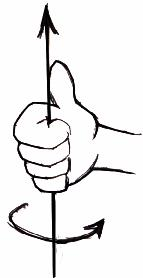The resultant motional E-field would be vertical to both and inwardly directed.

At the same time the current in the other half of the conductors in the coils is moving down and both the current and magnetic field follow the right-hand rule.The resulting motional E-field is again vertical to both and inwardly directed.

Thus, the resultant field intensity is double the intensity attributable to either one of the set of coil conductors taken singularly.

Expressed mathematically: E = ( B x V ) + ( -B x -V ) = 2 ( B x V )

Putting each bifilar element into each Coil, we get: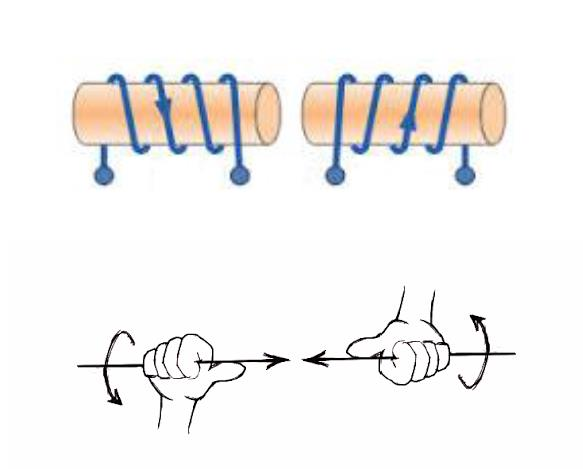Again, a step closer to achieving Floyd Sweet level of Energy Machine, perhaps. I think it depends on how open ones mind is to the possibilities?

October 10, 1871, Patent No. 119,825, Daniel McFarland Cook was right all along, we just weren't smart enough to figure it out!

Perhaps a little more that Speculation! Voltage is an absolute Requirement here and if the Change in B regulates V by so much we have not enough V to do anything with, then we have a problem.

I have many turns on my Coils for this reason. CSA is another method to reduce the turns.

Best wishes, stay safe and well My Friends,

Chris

Chris posted this 4 weeks ago

My Friends,

Continuing with Guessing is not going to be sufficient to make progress!

We all should know, by now, the Requirement for AU Machines are:

• Voltage
• and Current

on the Output! We all know the Equation for Power is: Power ( P ) = Voltage ( V ) x Current ( I ) x Cos(Theta Θ) and for Energy, Time ( t ) is Multiplied by this Product. P x t = Joules ( J ).

We cannot have a Current, without a Voltage!

I started a Thread Here, called: Calculating the Magnetic Field B, and then Voltage V, but it attracted very little interest!

Voltage is "Generated", which is predicted by Faradays Law Equation: E.M.F = -N dΦb / dt

I have given you all a Calculator, on the very First Tab, where we can use this Calculator to predict a Voltage for a given set of Parameters: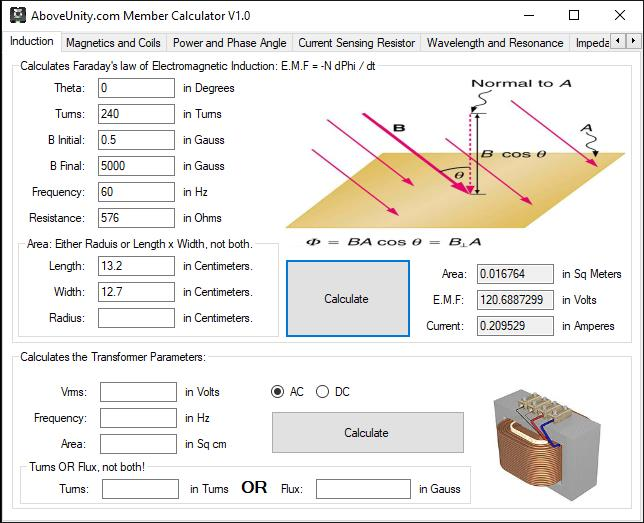Floyd Sweet had no more than 2 Inches, 5 Centimeters or 50 Millimeters of Coil Length and I believe he had each Coil stacked on top of the other! This means each Coil was no more than 25 Millimeters or 2.5 Centimeters!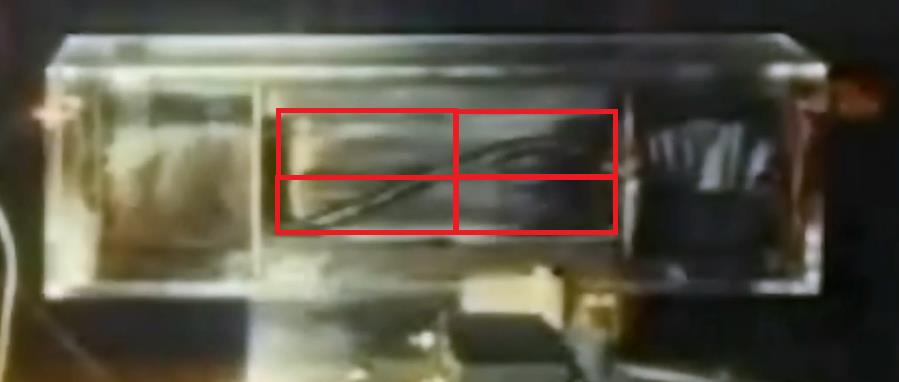Study this Image carefully: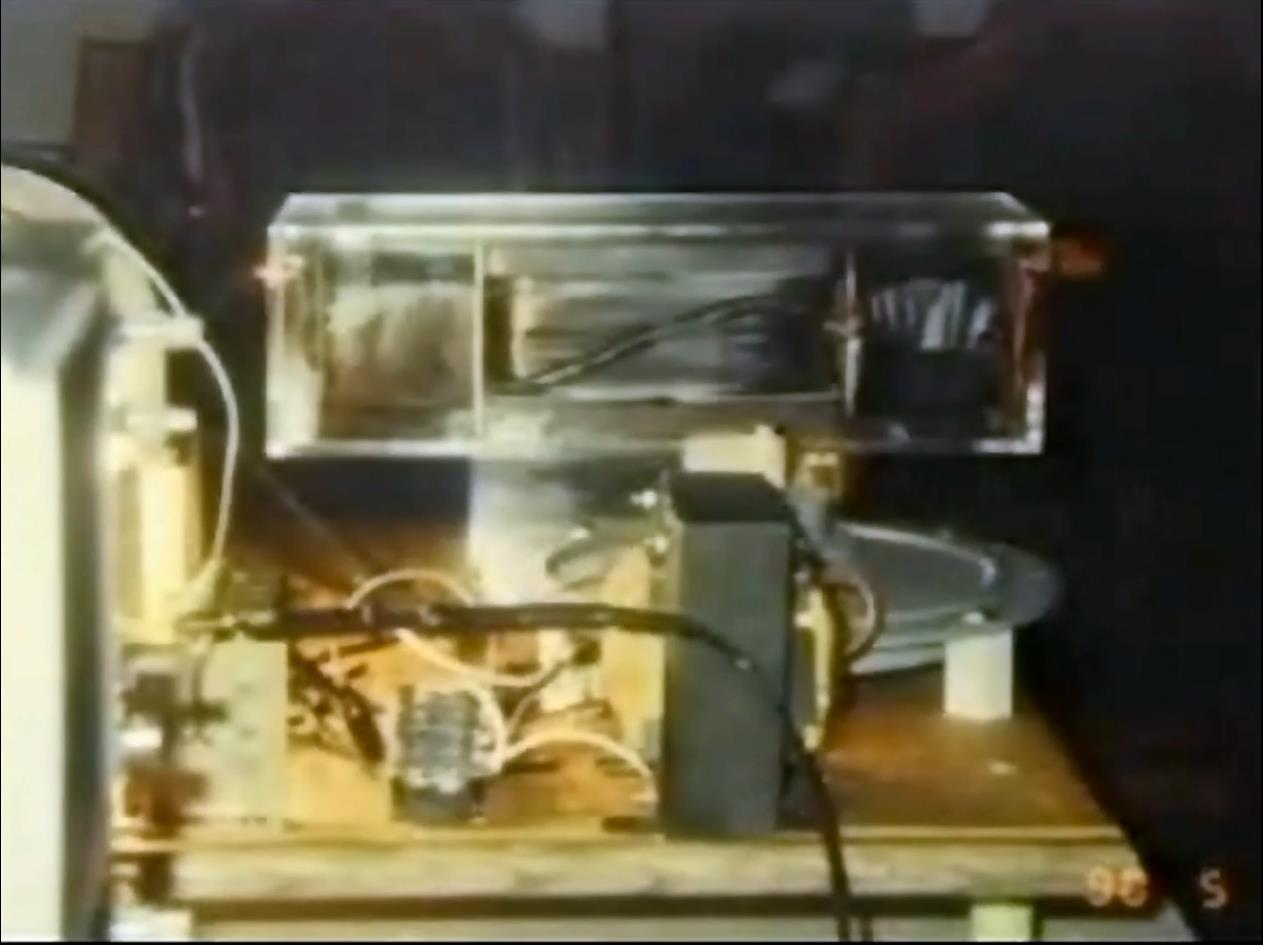Ref: Floyd Sweet VTA Generation 5.

Why would Floyd Sweet use such Short length in Coils?

Remember what Walt Rosenthal told us:

The VTA "likes" to always see a minimum load of 25 watts.

Ref: Walt Rosenthal

Well, Ohms Law is our friend here, we can calculate the Current: 0.20833 Amperes.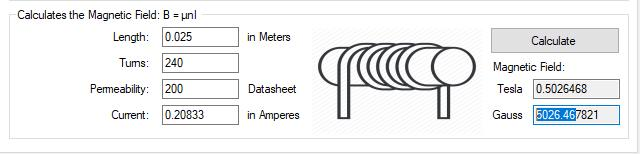Coil length ( l ) is what Increases the Magnetic Field ( B ) for the same Turns ( N ) and Current ( I ), Experiment with this, I gave you a Calculator on the Second Tab that uses Amperes Law to calculate the Coil's Magnetic Field ( B ), very easy and simple!

So, the question: "Why would Floyd Sweet use such Short length in Coils?", the answer is very simple! As the Magnetic Field ( B ) increases, we can Increase the Voltage ( V ) "Generated" for the same Turns ( ) and Current ( I )!

It makes sense that the Magnetic Field ( ) is as Density populated as possible for a given Area ( A ), and Changing in Time ( t ) as much as possible! Logical and Sensible!

NOTE: Most Transformers can operate, and are designed to Operate around 1.0 - 1.2 Tesla or 10,000.0 - 12,000.0 Gauss. This is a normal Operating Region.

Floyd Sweet was so far ahead of his time!

I think its time we start making Experiments a bit Smarter, stop guessing, making experiments more productive and more beneficial. Already we have totally left the Trolls in the Dust!

If Readers understand what I am saying here, the World will never be the same again!

Best Wishes,

Chris

Members Online:

No one online at the moment

Since 03.08.17 - Widget: 27.11.18
Recomended Protocol:MeasurementMeasurement BlockCurrent ObservationCurrent Sensing Resolution
Our Above Unity Machines:Romanian ZPMWistiti's Bucking Joule ThiefYo's Ferro-Magnetic ResonanceJagau's Non-linear ResonanceCD's Non-Inductive Coil RepNon-Inductive Coil ExperimentBaerndorfer's ExperimentCaptainloz's A-R-EFerrite at work
Start Here:Help with using the Forum.

More than anything else, your contributions to this forum are most important! We are trying to actively get all visitors involved, but we do only have a few main contributors, which are very much appreciated! If you would like to see more pages with more detailed experiments and answers, perhaps a contribution of another type maybe possible:

PayPal De-Platformed me!

They REFUSE to tell me why!

The content I am sharing is not only unique, but is changing the world as we know it! Please Support Us!

Perhaps a Bank Transfer?

• Bank: Bank of Queensland
• Name: Christopher R Sykes
• BSB: 124-001
• Account: 21580359

Thank You So Much!

Weeks High Earners:
The great Nikola Tesla:Ere many generations pass, our machinery will be driven by a power obtainable at any point of the universe. This idea is not novel. Men have been led to it long ago go by instinct or reason. It has been expressed in many ways, and in many places, in the history of old and new. We find it in the delightful myth of Antheus, who drives power from the earth; we find it among the subtle speculations of one of your splendid mathematicians, and in many hints and statements of thinkers of the present time. Throughout space there is energy. Is this energy static or kinetic? If static, our hopes are in vain; if kinetic - and this we know it is for certain - then it is a mere question of time when men will succeed in attaching their machinery to the very wheelwork of nature.

Experiments With Alternate Currents Of High Potential And High Frequency (February 1892).

Close